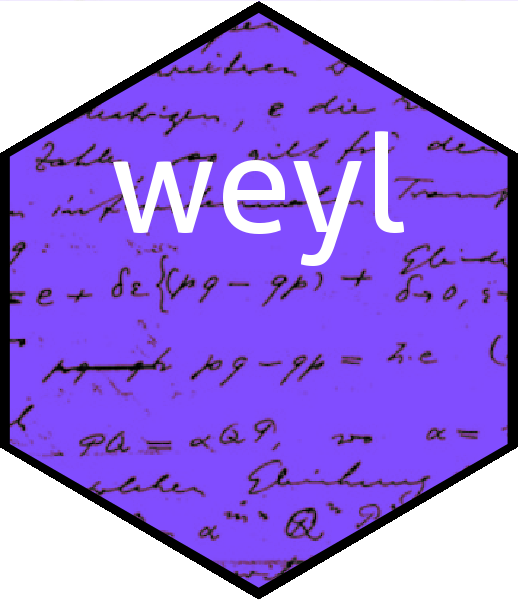To cite this work or the weyl package in publications please use Hankin (2022). In a very nice youtube video, Richard Borcherds discusses the fact that first-order differential operators do not quite commute, but their commutator is itself first-order; here I demonstrate Borcherds’s observations in the context of the weyl package. Symbolically, if

$D=\sum f_i\left(x_1,\dots,x_n\right)\frac{\partial}{\partial x_i}\qquad E=\sum g_i\left(x_1,\dots,x_n\right)\frac{\partial}{\partial x_i}$

where $$f_i=f_i\left(x_1,\dots,x_n\right)$$ and $$g_i=g_i\left(x_1,\dots,x_n\right)$$ are functions, then

$DE=\sum_{i,j}f_i\frac{\partial}{\partial x_i}\,g_i\frac{\partial}{\partial x_j} =\sum_{i,j}f_ig_j\frac{\partial}{\partial x_i}\frac{\partial}{\partial x_j} + f_i\frac{\partial g_j}{\partial x_i}\,\frac{\partial}{\partial x_j}$

$ED=\sum_{i,j}g_i\frac{\partial}{\partial x_i}\,f_i\frac{\partial}{\partial x_j} =\sum_{i,j}g_if_j\frac{\partial}{\partial x_j}\frac{\partial}{\partial x_i} + g_i\frac{\partial f_i}{\partial x_j}\,\frac{\partial}{\partial x_j}$

so $$E$$ and $$E$$ “nearly” commute, in the sense that $$ED-DE$$ is first order:

$DE-ED= \sum_{i,j}f_i\frac{\partial g_j}{\partial x_i}\,\frac{\partial}{\partial x_j}-g_i\frac{\partial f_i}{\partial x_j}\,\frac{\partial}{\partial x_j}$

Above we have used the fact that partial derivatives commute, which leads to the cancellation of the second-order terms. We can verify this using the weyl package:

D <- weyl(spray(cbind(matrix(sample(8),4,2),kronecker(diag(2),c(1,1))),1:4))
E <- weyl(spray(cbind(matrix(sample(8),4,2),kronecker(diag(2),c(1,1))),1:4))
F <- weyl(spray(cbind(matrix(sample(8),4,2),kronecker(diag(2),c(1,1))),1:4))
D
## A member of the Weyl algebra:
##   x  y dx dy     val
##   7  8  0  1  =    4
##   4  3  0  1  =    3
##   1  5  1  0  =    2
##   6  2  1  0  =    1

($$E$$ and $$F$$ are similar). Symbolically we would have

$D= \left( x^6y^2 + 2xy^5\right)\frac{\partial}{\partial x}+ \left(4x^7y^8 + 3x^4y^3\right)\frac{\partial}{\partial y}$

The package allows us to compose $$E$$ and $$D$$, although the composition is quite complicated:

summary(E*D)
## A spray object.  Summary of coefficients:
##
## a disord object with hash ab0b4d525d3ef5030fcdc229e07f50175ee9dc3f
##
##    Min. 1st Qu.  Median    Mean 3rd Qu.    Max.
##     1.0     4.0     8.5    20.5    22.0   128.0
##
##
## Representative selection of index and coefficients:
##
##   x  y dx dy     val
##  11  3  1  0  =    6
##   9 12  1  1  =   16
##   9  4  0  1  =   12
##   8  6  2  0  =    2
##  10  4  1  1  =    3
##  12  3  2  0  =    1

However, the Lie bracket, $$ED-DE$$, (.[E,D] in package idiom) is indeed first order:

.[E,D]
## A member of the Weyl algebra:
##   x  y dx dy     val
##   8 12  0  1  =   -8
##   4  9  1  0  =   30
##  15 14  0  1  =   16
##  12  9  0  1  =  -20
##   5  7  0  1  =   24
##   7  6  1  0  =    8
##  13  8  1  0  =   -4
##  14  8  1  0  =    8
##   8  7  0  1  =   -9
##  13  9  0  1  =  -32
##  10  3  1  0  =   -3
##   9 11  1  0  =    8
##   7  7  0  1  =  -18
##   2  9  1  0  =   -4
##   3 10  0  1  =  -18
##   9  6  1  0  =    6
##   6  6  1  0  =  -34
##   9  4  0  1  =   12
##  10 12  0  1  =   36

Above we see that each row is either 1 0 or 0 1, corresponding to either $$\partial/\partial x$$ or $$\partial/\partial y$$ respectively. Arguably this is easier to see with the other print method:

options(polyform = TRUE)
.[E,D]
## A member of the Weyl algebra:
## -8*x^8*y^12*dy +30*x^4*y^9*dx +16*x^15*y^14*dy -20*x^12*y^9*dy
## +24*x^5*y^7*dy +8*x^7*y^6*dx -4*x^13*y^8*dx +8*x^14*y^8*dx
## -9*x^8*y^7*dy -32*x^13*y^9*dy -3*x^10*y^3*dx +8*x^9*y^11*dx
## -18*x^7*y^7*dy -4*x^2*y^9*dx -18*x^3*y^10*dy +6*x^9*y^6*dx
## -34*x^6*y^6*dx +12*x^9*y^4*dy +36*x^10*y^12*dy
options(polyform = FALSE) # revert to default

We may verify Jacobi’s identity:

.[D,.[E,F]] + .[F,.[D,E]] + .[E,.[F,D]]
## A member of the Weyl algebra:
## empty sparse array with 4 columns

Borcherds goes on to consider the special case where the $$f_i$$ and $$g_i$$ are constant. In this case the operators commute (by repeated application of Schwarz’s theorem) and so their Lie bracket is identically zero. We can create constant operators easily:

(D <- as.weyl(spray(cbind(matrix(0,3,3),matrix(c(0,1,0,1,0,0,0,0,1),3,3,byrow=T)),1:3)))
## A member of the Weyl algebra:
##   x  y  z dx dy dz     val
##   0  0  0  0  0  1  =    3
##   0  0  0  1  0  0  =    2
##   0  0  0  0  1  0  =    1
(E <- as.weyl(spray(cbind(matrix(0,3,3),matrix(c(0,1,0,1,0,0,0,0,1),3,3,byrow=T)),5:7)))
## A member of the Weyl algebra:
##   x  y  z dx dy dz     val
##   0  0  0  0  0  1  =    7
##   0  0  0  1  0  0  =    6
##   0  0  0  0  1  0  =    5

(above, see how the first three columns of the index matrix are zero, corresponding to constant coefficients of the differential operator; symbolically $$D=2\frac{\partial}{\partial x}+\frac{\partial}{\partial y}+3\frac{\partial}{\partial z}$$ and $$E=6\frac{\partial}{\partial x}+5\frac{\partial}{\partial y}+7\frac{\partial}{\partial z}$$. And indeed, their Lie bracket vanishes:

.[D,E]
##  0

Hankin, R. K. S. 2022. “Quantum Algebra in R: The Weyl Package.” arXiv. https://doi.org/10.48550/ARXIV.2212.09230.